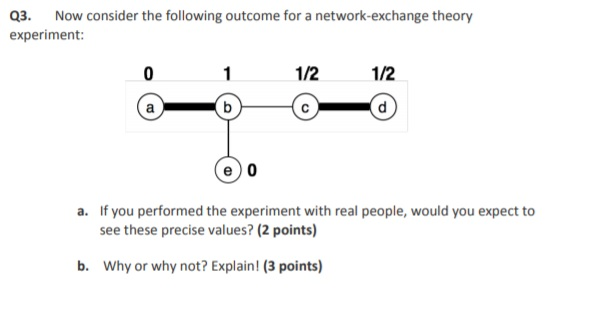# Q3. Now consider the following outcome for a network-exchange theory experiment: 1/2 1/2 a. If you performed the experi...Q3. Now consider the following outcome for a network-exchange theory experiment: 1/2 1/2 a. If you performed the experiment with real people, would you expect to see these precise values? (2 points) b. Why or why not? Explain! (3 points)

Solution: This is a five path network, with the node b occupying the intuitively strong position. The reason is that the nodes can engage in free-form negotiation in which units of values on edge can be divided. The node b can be able reach an agreement with all nodes, therefore if this network is deployed with real people, we can expect precise values.

Note : I have answered in brief based on the 3 points, comment me if you want a detailed explanation.

##### Add Answer of: Q3. Now consider the following outcome for a network-exchange theory experiment: 1/2 1/2 a. If you performed the experi...
More Homework Help Questions Additional questions in this topic.